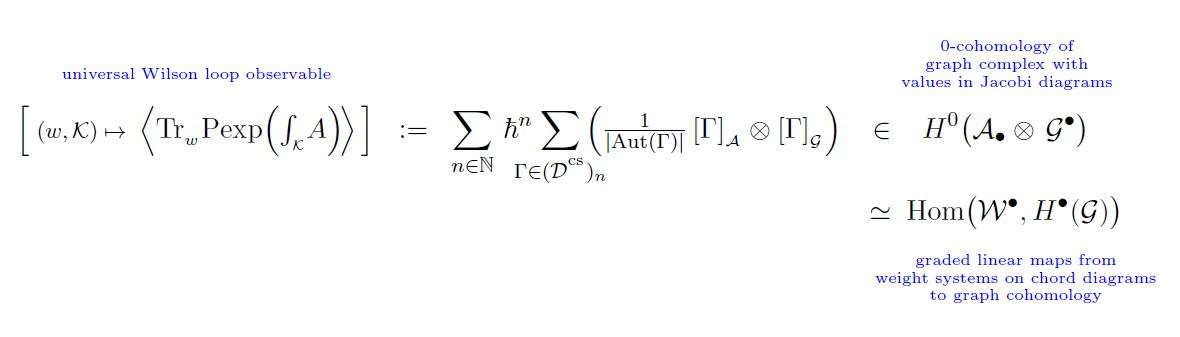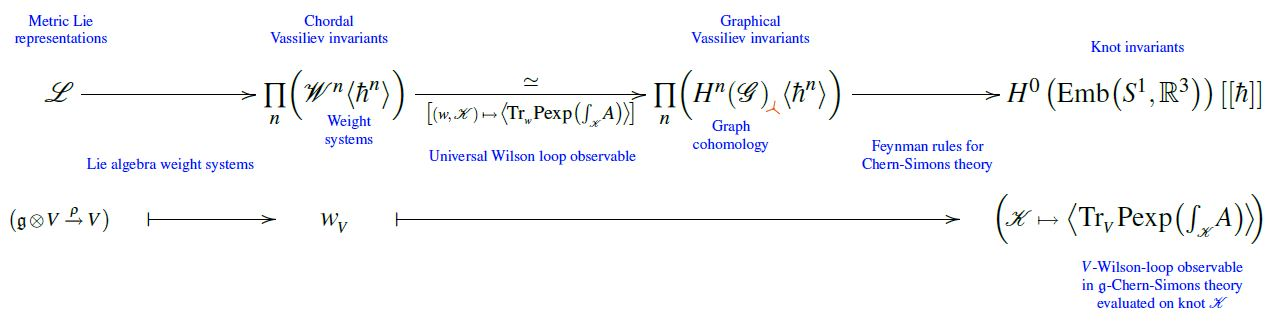# nLab universal Vassiliev invariant

Contents

### Context

#### Knot theory

knot theory

Examples/classes:

Types

knot invariants

Related concepts:

category: knot theory

# Contents

## Idea

A universal Vassiliev invariant is a Vassiliev knot invariant with coefficients in formal power series of Jacobi diagrams in Planck's constant $\hbar$ whose leading term in $\hbar$ on a given singular knot is its chord diagram.

By evaluating (framed) weight systems on the Jacobi diagram coefficients of a universal Vassiliev invariant it defines a function from framed weight systems on Jacobi diagrams, equivalently on round chord diagrams to actual (ground field-valued) Vassiliev knot invariants.

This construction in particular yields the proof that weight systems are the associated graded objects of Vassiliev invariants.

In principle there is a vector space of universal Vassiliev invariants, but all that appear in the literature tend to agree (BNGRT 97) and are identified (AF 96) with the un-traced Wilson loop observable of perturbative Chern-Simons theory:The main other known universal Vassiliev iunvariant is the Kontsevich integral (see Lescop 02 for the comparison).

## Ingredients

Write

(1)$\mathcal{D}^{cs} \;\in\; Set^{\mathbb{N}}$

for the graded set of isomorphism classes of trivalent framed knot graphs – Feynman diagrams for Chern-Simons theory in the presence of Wilson loops, called “Wilson graphs” AF 96, Section 1, slightly differing from the un-framed knot graphs in CCRL 02.

By definition, a graph $\Gamma \in \mathcal{D}^{cs}$ must have even number of vertices, and its degree is half that number (AF 96, (2.9))

$\Gamma \;\in\; \mathcal{D}^{cs}_{(\# Vert_\Gamma)/2} \,.$

For any $\Gamma \in \mathcal{D}^{cs}$ write

$Aut(\Gamma) \;\in\; Grp$

for its automorphism group, a finite group whose order we denote by

(2)$\left\vert Aut(\Gamma)\right\vert \;\in\; \mathbb{N} \,.$

Write

(3)$\mathcal{G}^\bullet \;\in\; Ch^\bullet(\mathbb{R})$

for the framed knot graph complex and

$H^\bullet(\mathcal{G})_3 \subset H^\bullet(\mathcal{G})$

for the sub-vector space of its cohomology spanned by cocycles made of trivalent graphs.

Write

(4)$\mathcal{A}_\bullet \;\coloneqq\; Span \left( \mathcal{D}^t \right)/(STU) \; \in \; Vect_\bullet(\mathbb{R})$

Also write

(5)$\array{ && \mathcal{D}^{cs} \\ & {}^{\mathllap{ [-]_{{}_{\mathcal{A}}} }} \swarrow && \searrow^{\mathrlap{ [-]_{\mathcal{G}} }} \\ \mathcal{A}_\bullet && && \mathcal{G}^\bullet }$

for the functions that send a graph to the defining basis vector that it represents in these vector spaces, respectively.

The space $\mathcal{A}_\bullet$ is the graded linear dual of the space of weight systems

$\mathcal{W}^\bullet \;\coloneqq\; (\mathcal{A}_\bullet)^\ast \,.$

Hence if we regard

$\mathcal{A}_\bullet \;=\; (\mathcal{A}^{-\bullet}, d= 0)$

as a cochain complex in non-positive degree with vanishing differential, then its tensor product of cochain complexes with the knot graph complex is the cochain complex whose closed elements are the graded linear maps from $\mathcal{W}^\bullet$ to the cochain cohomology $H^\bullet(GraphCplx)$ of the knot graph complex:

(6)$H^0 \big( \mathcal{A}_\bullet \otimes \mathcal{G}^\bullet \big) \;\simeq\; Hom \big( \mathcal{W}^\bullet , H^\bullet(\mathcal{G}) \big)$

## Statement

###### Proposition

The element

(7)$\left\langle Tr_{(-)} \text{P}\exp \left( \int_{(-)} A \right) \right\rangle \;\coloneqq\; \underset{ n \in \mathbb{N} }{\sum} \hbar^n \underset{ \Gamma \in \mathcal{D}^{cs}_n }{\sum} \left( \frac{1}{\left\vert \Gamma\right\vert} \, [\Gamma]_{\mathcal{A}} \otimes [\Gamma]_{\mathcal{G}} \right) \; \in \;\; \mathcal{A}_\bullet \otimes \mathcal{G}^\bullet$

(hence the sum over Feynman diagrams (1) of the tensor product of their images (5) in Jacobi diagrams modulo the STU-relations (4) and in the knot graph complex (3), respectively, weighted by the inverse order of their automorphism group (2) )

is closed

$d_{\mathcal{G}} \left\langle Tr_{(-)} \text{P}\exp \left( \int_{(-)} A \right) \right\rangle \;=\; 0$

This is AF 96, Theorem 1.

Hence (7) defines a cochain cohomology-class

\begin{aligned} \left\langle Tr_{(-)} \text{P}\exp \left( \int_{(-)} A \right) \right\rangle \;\coloneqq\; H^0 \big( \mathcal{A}_\bullet \otimes \mathcal{G}^\bullet \big) \\ & \;\simeq\; Hom \big( \mathcal{W}^\bullet , H^\bullet(\mathcal{G}) \big) \end{aligned}

and hence, by (6), it defines a graded linear function

$\mathcal{W}^\bullet \overset{ \left\langle Tr_{(-)} \text{P}\exp \left( \int_{(-)} A \right) \right\rangle }{ \longrightarrow } H^\bullet(\mathcal{G})_3 \hookrightarrow H^\bullet(\mathcal{G})$

from weight systems on Jacobi diagrams (equivalently on round chord diagrams) to the cochain cohomology of the framed knot graph complex spanned by trivalent graphs.

According to CCRL 02, Prop. 7.6 this map is a bijection.

To see this, use 1) AF 96, Theorem 5, Condition U2 to find that the map is an injection, and 2) the fact that weight systems are associated graded of Vassiliev invariants.

### General

Textbook account

Original articles:

Review:

### Comparison

Comparison between the two main universal Vassiliev invariants: the Kontsevich integral and the Wilson loop observable of perturbatively quantized Chern-Simons theory:

### Special values

Computation of the perturbative Chern-Simons Wilson loop observable (universal Vassiliev invariant) of the unknot (“Wheels theorem”):

following